## 深入理解 JavaScript 正则表达式的特性与最佳实践

JavaScript 的正则表达式借鉴自 Perl。

JavaScript 正则表达式的语法对 Perl 进行了改进与扩张。但它的书写规则非常复杂，所以只有对正则表达式有着透彻的理解，才能写好它。为了缓解这个问题，这里对它的规则进行了简化，尽量减少出错的可能。这是值得的。

# 1 示例

var parse_url = /^(?:([A-Za-z]+):)?(/{0,3})([0-9.-A-Za-z]+)(?::(d+))?(?:/([^?#]*))?(?:?([^#]*))?(?:#(.*))?\$/;

var url = "http://www.ora.com:80/goodparts?q#fragment";

var result = parse_url.exec(url);

var names = ['url','scheme','slash','host','port','path','query','hash'];

var blanks = ' ';

var i;

for (i = 0; i < names.length; i += 1) {

console.log(names[i] + ':' + blanks.substring(names[i].length),result[i]);

}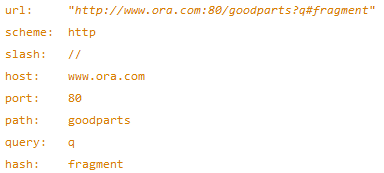^ 字符表示这个字符串的开始部分，只匹配那些从开头就像 URL 的字符串：

^(?:([A-Za-z]+):)?

(/{0,3})

([0-9.-A-Za-z]+)

(?::(d+))?

(?:/([^?#]*))?

(?:?([^#]*))?

(?:#(.*))?

\$

/**

* 匹配数字

*/

var parse_number = /^-?d+(?:.d*)?(?:e[+-]?d+)?\$/i;

var test = function (num) {

console.log(parse_number.test(num));

};

test('1');//true

test('number');//false

test('98.6');//true'

test('23.2138.23');//false

test('123.2E-38');//true

test('123.2D-38');//false

/^ \$/i

i 标识表示匹配字母时，忽略大小写。数字中可能出现的字母是 e，所以我们希望它既能匹配 e，也能匹配 E。

-?

d+

d 的含义与 [0-9] 一样，它们都是匹配一个数字。后缀 + 匹配一个或多个数字。

(?:.d*)?

(?: …) 表示一个可选的非捕获型分组。子所以使用非捕获型分组，是因为捕获型分组会有性能上的损失。这个分组会匹配 0 个或多个数字的小数点。

(?:e[+-]?d+)?

# 2 结构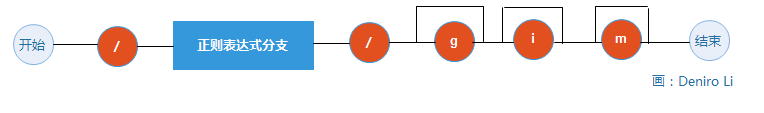RegExp 可以设置 3 个标识：

g

i

m

var parse_number = /^-?d+(?:.d*)?(?:e[+-]?d+)?\$/i;

RegExp 对象的属性：

global

ignoreCase

lastIndex

multiline

source

# 3 正则表达式的元素

## 3.1 分支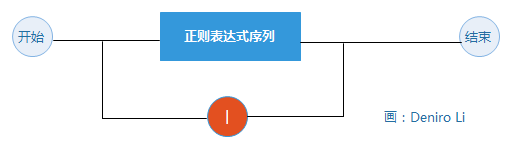"into".match(/in|int/)

## 3.2 序列## 3.3 因子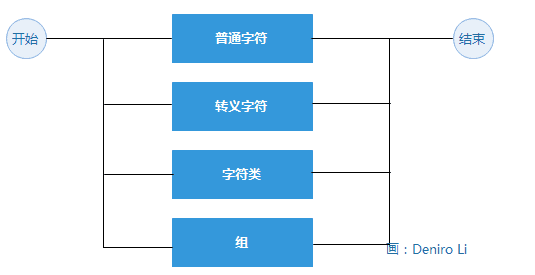/ [ ] ( ) { } ? + * | . ^ \$

f

n

r

u

d

s

S

w

W

b

1

## 3.5 分组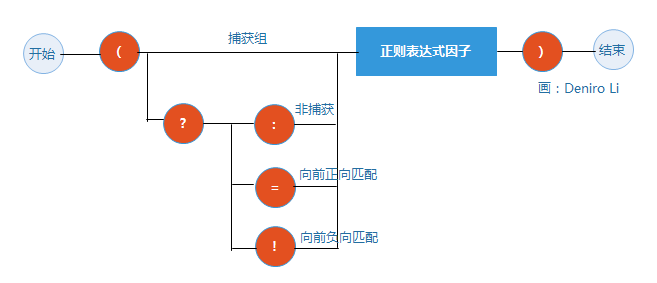## 3.6 字符集

1. 指定字符范围。

2. 对类求反。如果 [ 后的第一个字符是 ^，那就会排除这些指定的字符。

- / [ ] ^

## 3.8 量词

/www/ 等同于 /w{3}/

{3,6} 匹配 3,4,5 或 6 次

{3,} 匹配 3 次或更多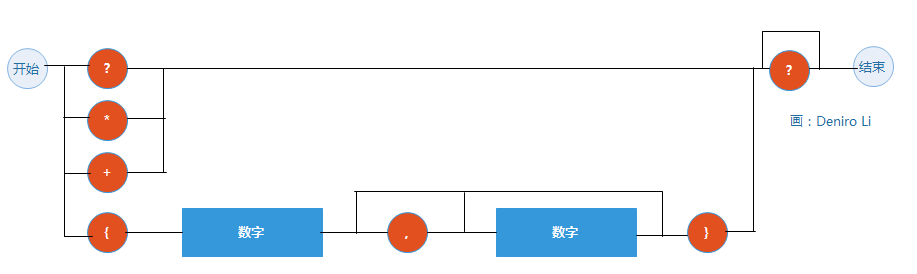? 相当于 {0,1}

* 相当于 {0,}

+ 相当于 {1,}

0人推荐

#### 推荐文章

•JavaScript

•JavaScript

•JavaScript

•JavaScript

•JavaScript

•JavaScript

•JavaScript

•JavaScript